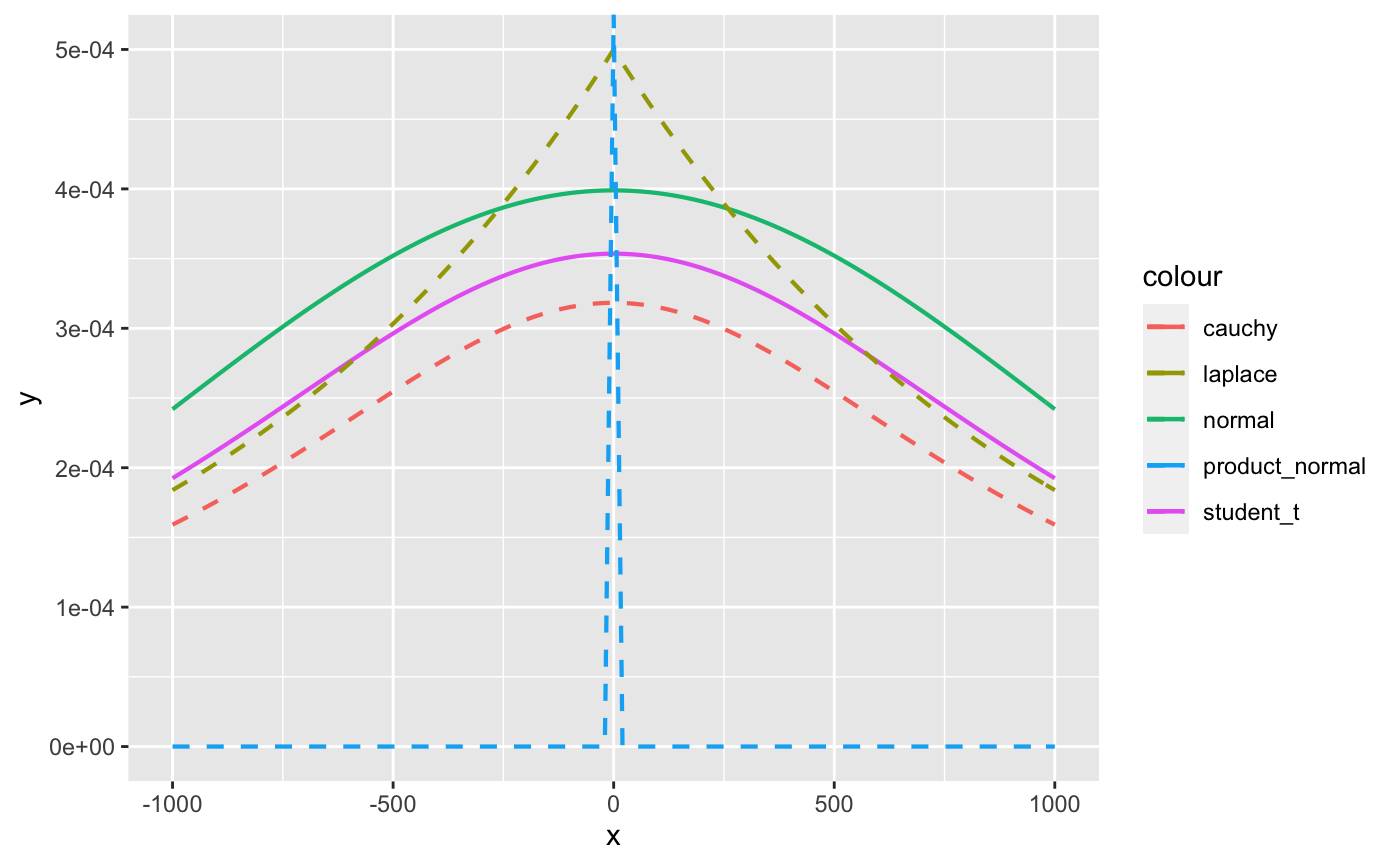The functions described on this page are used to specify the prior-related arguments of the various modeling functions in the rstanarm package (to view the priors used for an existing model see prior_summary).

The default priors used in the various rstanarm modeling functions are intended to be weakly informative in that they provide moderate regularization and help stabilize computation. For many applications the defaults will perform well, but prudent use of more informative priors is encouraged. Uniform prior distributions are possible (e.g. by setting stan_glm's prior argument to NULL) but, unless the data is very strong, they are not recommended and are not non-informative, giving the same probability mass to implausible values as plausible ones.

More information on priors is available in the vignette Prior Distributions for rstanarm Models as well as the vignettes for the various modeling functions. For details on the priors used for multilevel models in particular see the vignette Estimating Generalized (Non-)Linear Models with Group-Specific Terms with rstanarm and also the Covariance matrices section lower down on this page.

normal(location = 0, scale = NULL, autoscale = FALSE)

student_t(df = 1, location = 0, scale = NULL, autoscale = FALSE)

cauchy(location = 0, scale = NULL, autoscale = FALSE)

hs(df = 1, global_df = 1, global_scale = 0.01, slab_df = 4, slab_scale = 2.5)

hs_plus(
df1 = 1,
df2 = 1,
global_df = 1,
global_scale = 0.01,
slab_df = 4,
slab_scale = 2.5
)

laplace(location = 0, scale = NULL, autoscale = FALSE)

lasso(df = 1, location = 0, scale = NULL, autoscale = FALSE)

product_normal(df = 2, location = 0, scale = 1)

exponential(rate = 1, autoscale = FALSE)

decov(regularization = 1, concentration = 1, shape = 1, scale = 1)

lkj(regularization = 1, scale = 10, df = 1, autoscale = TRUE)

dirichlet(concentration = 1)

R2(location = NULL, what = c("mode", "mean", "median", "log"))

default_prior_intercept(family)

default_prior_coef(family)

## Arguments

location Prior location. In most cases, this is the prior mean, but for cauchy (which is equivalent to student_t with df=1), the mean does not exist and location is the prior median. The default value is $$0$$, except for R2 which has no default value for location. For R2, location pertains to the prior location of the $$R^2$$ under a Beta distribution, but the interpretation of the location parameter depends on the specified value of the what argument (see the R2 family section in Details). Prior scale. The default depends on the family (see Details). If TRUE then the scales of the priors on the intercept and regression coefficients may be additionally modified internally by rstanarm in the following cases. First, for Gaussian models only, the prior scales for the intercept, coefficients, and the auxiliary parameter sigma (error standard deviation) are multiplied by sd(y). Additionally --- not only for Gaussian models --- if the QR argument to the model fitting function (e.g. stan_glm) is FALSE then we also divide the prior scale(s) by sd(x). Prior autoscaling is also discussed in the vignette Prior Distributions for rstanarm Models Prior degrees of freedom. The default is $$1$$ for student_t, in which case it is equivalent to cauchy. For the hierarchical shrinkage priors (hs and hs_plus) the degrees of freedom parameter(s) default to $$1$$. For the product_normal prior, the degrees of freedom parameter must be an integer (vector) that is at least $$2$$ (the default). Optional arguments for the hierarchical shrinkage priors. See the Hierarchical shrinkage family section below. Prior rate for the exponential distribution. Defaults to 1. For the exponential distribution, the rate parameter is the reciprocal of the mean. Exponent for an LKJ prior on the correlation matrix in the decov or lkj prior. The default is $$1$$, implying a joint uniform prior. Concentration parameter for a symmetric Dirichlet distribution. The default is $$1$$, implying a joint uniform prior. Shape parameter for a gamma prior on the scale parameter in the decov prior. If shape and scale are both $$1$$ (the default) then the gamma prior simplifies to the unit-exponential distribution. A character string among 'mode' (the default), 'mean', 'median', or 'log' indicating how the location parameter is interpreted in the LKJ case. If 'log', then location is interpreted as the expected logarithm of the $$R^2$$ under a Beta distribution. Otherwise, location is interpreted as the what of the $$R^2$$ under a Beta distribution. If the number of predictors is less than or equal to two, the mode of this Beta distribution does not exist and an error will prompt the user to specify another choice for what. Not currently used.

## Value

A named list to be used internally by the rstanarm model fitting functions.

## Details

The details depend on the family of the prior being used:

### Student t family

Family members:

• normal(location, scale)

• student_t(df, location, scale)

• cauchy(location, scale)

Each of these functions also takes an argument autoscale.

For the prior distribution for the intercept, location, scale, and df should be scalars. For the prior for the other coefficients they can either be vectors of length equal to the number of coefficients (not including the intercept), or they can be scalars, in which case they will be recycled to the appropriate length. As the degrees of freedom approaches infinity, the Student t distribution approaches the normal distribution and if the degrees of freedom are one, then the Student t distribution is the Cauchy distribution.

If scale is not specified it will default to $$2.5$$, unless the probit link function is used, in which case these defaults are scaled by a factor of dnorm(0)/dlogis(0), which is roughly $$1.6$$.

If the autoscale argument is TRUE, then the scales will be further adjusted as described above in the documentation of the autoscale argument in the Arguments section.

### Hierarchical shrinkage family

Family members:

• hs(df, global_df, global_scale, slab_df, slab_scale)

• hs_plus(df1, df2, global_df, global_scale, slab_df, slab_scale)

The hierarchical shrinkage priors are normal with a mean of zero and a standard deviation that is also a random variable. The traditional hierarchical shrinkage prior utilizes a standard deviation that is distributed half Cauchy with a median of zero and a scale parameter that is also half Cauchy. This is called the "horseshoe prior". The hierarchical shrinkage (hs) prior in the rstanarm package instead utilizes a regularized horseshoe prior, as described by Piironen and Vehtari (2017), which recommends setting the global_scale argument equal to the ratio of the expected number of non-zero coefficients to the expected number of zero coefficients, divided by the square root of the number of observations.

The hierarhical shrinkpage plus (hs_plus) prior is similar except that the standard deviation that is distributed as the product of two independent half Cauchy parameters that are each scaled in a similar way to the hs prior.

The hierarchical shrinkage priors have very tall modes and very fat tails. Consequently, they tend to produce posterior distributions that are very concentrated near zero, unless the predictor has a strong influence on the outcome, in which case the prior has little influence. Hierarchical shrinkage priors often require you to increase the adapt_delta tuning parameter in order to diminish the number of divergent transitions. For more details on tuning parameters and divergent transitions see the Troubleshooting section of the How to Use the rstanarm Package vignette.

### Laplace family

Family members:

• laplace(location, scale)

• lasso(df, location, scale)

Each of these functions also takes an argument autoscale.

The Laplace distribution is also known as the double-exponential distribution. It is a symmetric distribution with a sharp peak at its mean / median / mode and fairly long tails. This distribution can be motivated as a scale mixture of normal distributions and the remarks above about the normal distribution apply here as well.

The lasso approach to supervised learning can be expressed as finding the posterior mode when the likelihood is Gaussian and the priors on the coefficients have independent Laplace distributions. It is commonplace in supervised learning to choose the tuning parameter by cross-validation, whereas a more Bayesian approach would be to place a prior on “it”, or rather its reciprocal in our case (i.e. smaller values correspond to more shrinkage toward the prior location vector). We use a chi-square prior with degrees of freedom equal to that specified in the call to lasso or, by default, 1. The expectation of a chi-square random variable is equal to this degrees of freedom and the mode is equal to the degrees of freedom minus 2, if this difference is positive.

It is also common in supervised learning to standardize the predictors before training the model. We do not recommend doing so. Instead, it is better to specify autoscale = TRUE, which will adjust the scales of the priors according to the dispersion in the variables. See the documentation of the autoscale argument above and also the prior_summary page for more information.

### Product-normal family

Family members:

• product_normal(df, location, scale)

The product-normal distribution is the product of at least two independent normal variates each with mean zero, shifted by the location parameter. It can be shown that the density of a product-normal variate is symmetric and infinite at location, so this prior resembles a “spike-and-slab” prior for sufficiently large values of the scale parameter. For better or for worse, this prior may be appropriate when it is strongly believed (by someone) that a regression coefficient “is” equal to the location, parameter even though no true Bayesian would specify such a prior.

Each element of df must be an integer of at least $$2$$ because these “degrees of freedom” are interpreted as the number of normal variates being multiplied and then shifted by location to yield the regression coefficient. Higher degrees of freedom produce a sharper spike at location.

Each element of scale must be a non-negative real number that is interpreted as the standard deviation of the normal variates being multiplied and then shifted by location to yield the regression coefficient. In other words, the elements of scale may differ, but the k-th standard deviation is presumed to hold for all the normal deviates that are multiplied together and shifted by the k-th element of location to yield the k-th regression coefficient. The elements of scale are not the prior standard deviations of the regression coefficients. The prior variance of the regression coefficients is equal to the scale raised to the power of $$2$$ times the corresponding element of df. Thus, larger values of scale put more prior volume on values of the regression coefficient that are far from zero.

### Dirichlet family

Family members:

• dirichlet(concentration)

The Dirichlet distribution is a multivariate generalization of the beta distribution. It is perhaps the easiest prior distribution to specify because the concentration parameters can be interpreted as prior counts (although they need not be integers) of a multinomial random variable.

The Dirichlet distribution is used in stan_polr for an implicit prior on the cutpoints in an ordinal regression model. More specifically, the Dirichlet prior pertains to the prior probability of observing each category of the ordinal outcome when the predictors are at their sample means. Given these prior probabilities, it is straightforward to add them to form cumulative probabilities and then use an inverse CDF transformation of the cumulative probabilities to define the cutpoints.

If a scalar is passed to the concentration argument of the dirichlet function, then it is replicated to the appropriate length and the Dirichlet distribution is symmetric. If concentration is a vector and all elements are $$1$$, then the Dirichlet distribution is jointly uniform. If all concentration parameters are equal but greater than $$1$$ then the prior mode is that the categories are equiprobable, and the larger the value of the identical concentration parameters, the more sharply peaked the distribution is at the mode. The elements in concentration can also be given different values to represent that not all outcome categories are a priori equiprobable.

### Covariance matrices

Family members:

• decov(regularization, concentration, shape, scale)

• lkj(regularization, scale, df)

(Also see vignette for stan_glmer, Estimating Generalized (Non-)Linear Models with Group-Specific Terms with rstanarm)

Covariance matrices are decomposed into correlation matrices and variances. The variances are in turn decomposed into the product of a simplex vector and the trace of the matrix. Finally, the trace is the product of the order of the matrix and the square of a scale parameter. This prior on a covariance matrix is represented by the decov function.

The prior for a correlation matrix is called LKJ whose density is proportional to the determinant of the correlation matrix raised to the power of a positive regularization parameter minus one. If regularization = 1 (the default), then this prior is jointly uniform over all correlation matrices of that size. If regularization > 1, then the identity matrix is the mode and in the unlikely case that regularization < 1, the identity matrix is the trough.

The trace of a covariance matrix is equal to the sum of the variances. We set the trace equal to the product of the order of the covariance matrix and the square of a positive scale parameter. The particular variances are set equal to the product of a simplex vector --- which is non-negative and sums to $$1$$ --- and the scalar trace. In other words, each element of the simplex vector represents the proportion of the trace attributable to the corresponding variable.

A symmetric Dirichlet prior is used for the simplex vector, which has a single (positive) concentration parameter, which defaults to $$1$$ and implies that the prior is jointly uniform over the space of simplex vectors of that size. If concentration > 1, then the prior mode corresponds to all variables having the same (proportion of total) variance, which can be used to ensure the the posterior variances are not zero. As the concentration parameter approaches infinity, this mode becomes more pronounced. In the unlikely case that concentration < 1, the variances are more polarized.

If all the variables were multiplied by a number, the trace of their covariance matrix would increase by that number squared. Thus, it is reasonable to use a scale-invariant prior distribution for the positive scale parameter, and in this case we utilize a Gamma distribution, whose shape and scale are both $$1$$ by default, implying a unit-exponential distribution. Set the shape hyperparameter to some value greater than $$1$$ to ensure that the posterior trace is not zero.

If regularization, concentration, shape and / or scale are positive scalars, then they are recycled to the appropriate length. Otherwise, each can be a positive vector of the appropriate length, but the appropriate length depends on the number of covariance matrices in the model and their sizes. A one-by-one covariance matrix is just a variance and thus does not have regularization or concentration parameters, but does have shape and scale parameters for the prior standard deviation of that variable.

Note that for stan_mvmer and stan_jm models an additional prior distribution is provided through the lkj function. This prior is in fact currently used as the default for those modelling functions (although decov is still available as an option if the user wishes to specify it through the prior_covariance argument). The lkj prior uses the same decomposition of the covariance matrices into correlation matrices and variances, however, the variances are not further decomposed into a simplex vector and the trace; instead the standard deviations (square root of the variances) for each of the group specific parameters are given a half Student t distribution with the scale and df parameters specified through the scale and df arguments to the lkj function. The scale parameter default is 10 which is then autoscaled, whilst the df parameter default is 1 (therefore equivalent to a half Cauchy prior distribution for the standard deviation of each group specific parameter). This prior generally leads to similar results as the decov prior, but it is also likely to be **less** diffuse compared with the decov prior; therefore it sometimes seems to lead to faster estimation times, hence why it has been chosen as the default prior for stan_mvmer and stan_jm where estimation times can be long.

### R2 family

Family members:

• R2(location, what)

The stan_lm, stan_aov, and stan_polr functions allow the user to utilize a function called R2 to convey prior information about all the parameters. This prior hinges on prior beliefs about the location of $$R^2$$, the proportion of variance in the outcome attributable to the predictors, which has a Beta prior with first shape hyperparameter equal to half the number of predictors and second shape hyperparameter free. By specifying what to be the prior mode (the default), mean, median, or expected log of $$R^2$$, the second shape parameter for this Beta distribution is determined internally. If what = 'log', location should be a negative scalar; otherwise it should be a scalar on the $$(0,1)$$ interval.

For example, if $$R^2 = 0.5$$, then the mode, mean, and median of the Beta distribution are all the same and thus the second shape parameter is also equal to half the number of predictors. The second shape parameter of the Beta distribution is actually the same as the shape parameter in the LKJ prior for a correlation matrix described in the previous subsection. Thus, the smaller is $$R^2$$, the larger is the shape parameter, the smaller are the prior correlations among the outcome and predictor variables, and the more concentrated near zero is the prior density for the regression coefficients. Hence, the prior on the coefficients is regularizing and should yield a posterior distribution with good out-of-sample predictions if the prior location of $$R^2$$ is specified in a reasonable fashion.

Gelman, A., Carlin, J. B., Stern, H. S., Dunson, D. B., Vehtari, A., and Rubin, D. B. (2013). Bayesian Data Analysis. Chapman & Hall/CRC Press, London, third edition. http://stat.columbia.edu/~gelman/book/

Gelman, A., Jakulin, A., Pittau, M. G., and Su, Y. (2008). A weakly informative default prior distribution for logistic and other regression models. Annals of Applied Statistics. 2(4), 1360--1383.

Piironen, J., and Vehtari, A. (2017). Sparsity information and regularization in the horseshoe and other shrinkage priors. https://arxiv.org/abs/1707.01694

Stan Development Team. Stan Modeling Language Users Guide and Reference Manual. https://mc-stan.org/users/documentation/.

The various vignettes for the rstanarm package also discuss and demonstrate the use of some of the supported prior distributions.

## Examples

fmla <- mpg ~ wt + qsec + drat + am

# Draw from prior predictive distribution (by setting prior_PD = TRUE)
prior_pred_fit <- stan_glm(fmla, data = mtcars, prior_PD = TRUE,
chains = 1, seed = 12345, iter = 250, # for speed only
prior = student_t(df = 4, 0, 2.5),
prior_intercept = cauchy(0,10),
prior_aux = exponential(1/2))#>
#> SAMPLING FOR MODEL 'continuous' NOW (CHAIN 1).
#> Chain 1:
#> Chain 1: Gradient evaluation took 2.2e-05 seconds
#> Chain 1: 1000 transitions using 10 leapfrog steps per transition would take 0.22 seconds.
#> Chain 1:
#> Chain 1:
#> Chain 1: WARNING: There aren't enough warmup iterations to fit the
#> Chain 1:          three stages of adaptation as currently configured.
#> Chain 1:          Reducing each adaptation stage to 15%/75%/10% of
#> Chain 1:          the given number of warmup iterations:
#> Chain 1:            init_buffer = 18
#> Chain 1:            adapt_window = 95
#> Chain 1:            term_buffer = 12
#> Chain 1:
#> Chain 1: Iteration:   1 / 250 [  0%]  (Warmup)
#> Chain 1: Iteration:  25 / 250 [ 10%]  (Warmup)
#> Chain 1: Iteration:  50 / 250 [ 20%]  (Warmup)
#> Chain 1: Iteration:  75 / 250 [ 30%]  (Warmup)
#> Chain 1: Iteration: 100 / 250 [ 40%]  (Warmup)
#> Chain 1: Iteration: 125 / 250 [ 50%]  (Warmup)
#> Chain 1: Iteration: 126 / 250 [ 50%]  (Sampling)
#> Chain 1: Iteration: 150 / 250 [ 60%]  (Sampling)
#> Chain 1: Iteration: 175 / 250 [ 70%]  (Sampling)
#> Chain 1: Iteration: 200 / 250 [ 80%]  (Sampling)
#> Chain 1: Iteration: 225 / 250 [ 90%]  (Sampling)
#> Chain 1: Iteration: 250 / 250 [100%]  (Sampling)
#> Chain 1:
#> Chain 1:  Elapsed Time: 0.02027 seconds (Warm-up)
#> Chain 1:                0.019899 seconds (Sampling)
#> Chain 1:                0.040169 seconds (Total)
#> Chain 1: #> Warning: The largest R-hat is 1.14, indicating chains have not mixed.
#> Running the chains for more iterations may help. See
#> http://mc-stan.org/misc/warnings.html#r-hat#> Warning: Bulk Effective Samples Size (ESS) is too low, indicating posterior means and medians may be unreliable.
#> Running the chains for more iterations may help. See
#> http://mc-stan.org/misc/warnings.html#bulk-ess#> Warning: Tail Effective Samples Size (ESS) is too low, indicating posterior variances and tail quantiles may be unreliable.
#> Running the chains for more iterations may help. See
#> http://mc-stan.org/misc/warnings.html#tail-essplot(prior_pred_fit, "hist")#> stat_bin() using bins = 30. Pick better value with binwidth.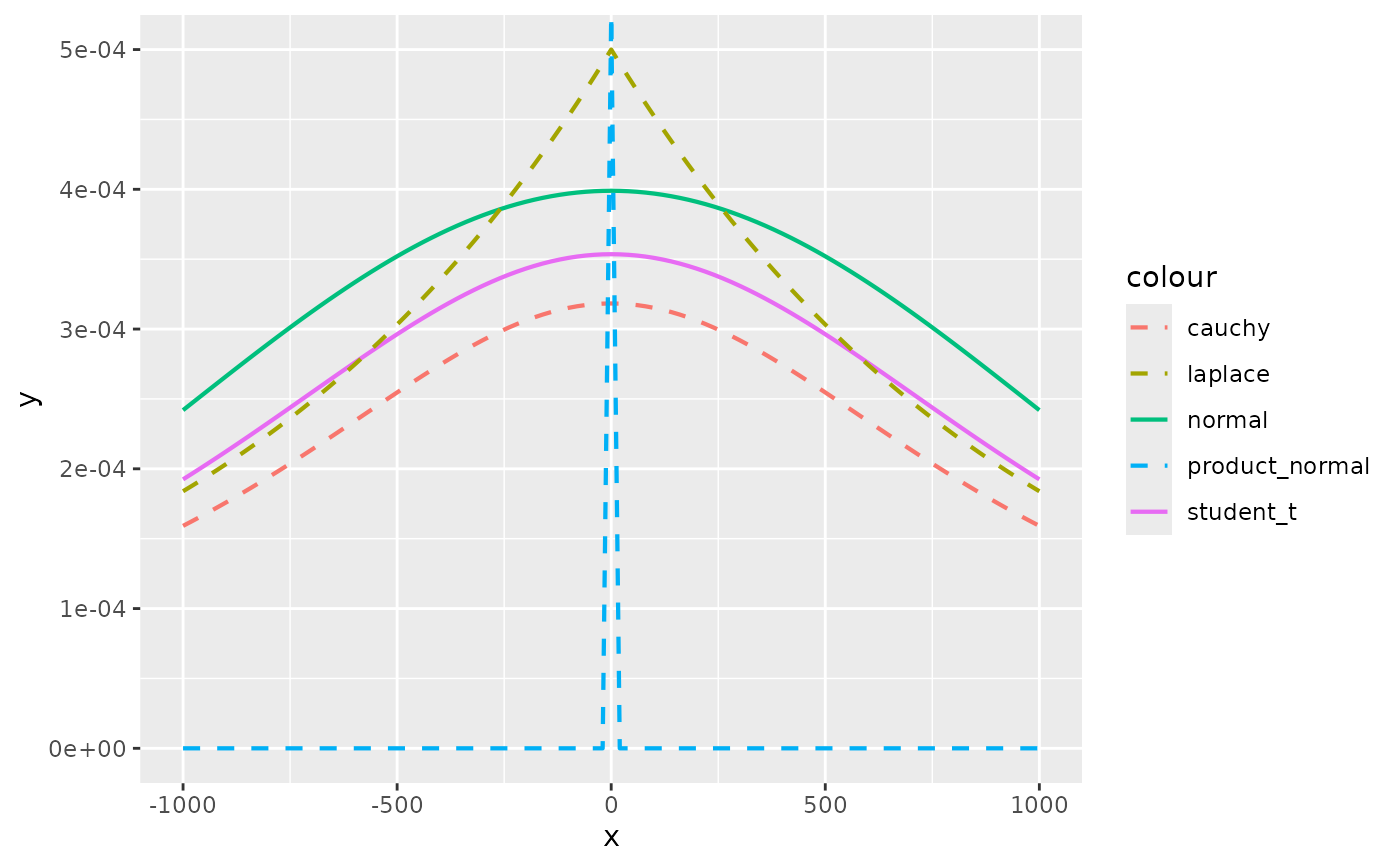# \donttest{
# Can assign priors to names
N05 <- normal(0, 5)
fit <- stan_glm(fmla, data = mtcars, prior = N05, prior_intercept = N05)#>
#> SAMPLING FOR MODEL 'continuous' NOW (CHAIN 1).
#> Chain 1:
#> Chain 1: Gradient evaluation took 2.1e-05 seconds
#> Chain 1: 1000 transitions using 10 leapfrog steps per transition would take 0.21 seconds.
#> Chain 1:
#> Chain 1:
#> Chain 1: Iteration:    1 / 2000 [  0%]  (Warmup)
#> Chain 1: Iteration:  200 / 2000 [ 10%]  (Warmup)
#> Chain 1: Iteration:  400 / 2000 [ 20%]  (Warmup)
#> Chain 1: Iteration:  600 / 2000 [ 30%]  (Warmup)
#> Chain 1: Iteration:  800 / 2000 [ 40%]  (Warmup)
#> Chain 1: Iteration: 1000 / 2000 [ 50%]  (Warmup)
#> Chain 1: Iteration: 1001 / 2000 [ 50%]  (Sampling)
#> Chain 1: Iteration: 1200 / 2000 [ 60%]  (Sampling)
#> Chain 1: Iteration: 1400 / 2000 [ 70%]  (Sampling)
#> Chain 1: Iteration: 1600 / 2000 [ 80%]  (Sampling)
#> Chain 1: Iteration: 1800 / 2000 [ 90%]  (Sampling)
#> Chain 1: Iteration: 2000 / 2000 [100%]  (Sampling)
#> Chain 1:
#> Chain 1:  Elapsed Time: 0.066157 seconds (Warm-up)
#> Chain 1:                0.059846 seconds (Sampling)
#> Chain 1:                0.126003 seconds (Total)
#> Chain 1:
#>
#> SAMPLING FOR MODEL 'continuous' NOW (CHAIN 2).
#> Chain 2:
#> Chain 2: Gradient evaluation took 1.4e-05 seconds
#> Chain 2: 1000 transitions using 10 leapfrog steps per transition would take 0.14 seconds.
#> Chain 2:
#> Chain 2:
#> Chain 2: Iteration:    1 / 2000 [  0%]  (Warmup)
#> Chain 2: Iteration:  200 / 2000 [ 10%]  (Warmup)
#> Chain 2: Iteration:  400 / 2000 [ 20%]  (Warmup)
#> Chain 2: Iteration:  600 / 2000 [ 30%]  (Warmup)
#> Chain 2: Iteration:  800 / 2000 [ 40%]  (Warmup)
#> Chain 2: Iteration: 1000 / 2000 [ 50%]  (Warmup)
#> Chain 2: Iteration: 1001 / 2000 [ 50%]  (Sampling)
#> Chain 2: Iteration: 1200 / 2000 [ 60%]  (Sampling)
#> Chain 2: Iteration: 1400 / 2000 [ 70%]  (Sampling)
#> Chain 2: Iteration: 1600 / 2000 [ 80%]  (Sampling)
#> Chain 2: Iteration: 1800 / 2000 [ 90%]  (Sampling)
#> Chain 2: Iteration: 2000 / 2000 [100%]  (Sampling)
#> Chain 2:
#> Chain 2:  Elapsed Time: 0.066193 seconds (Warm-up)
#> Chain 2:                0.08006 seconds (Sampling)
#> Chain 2:                0.146253 seconds (Total)
#> Chain 2:
#>
#> SAMPLING FOR MODEL 'continuous' NOW (CHAIN 3).
#> Chain 3:
#> Chain 3: Gradient evaluation took 1.6e-05 seconds
#> Chain 3: 1000 transitions using 10 leapfrog steps per transition would take 0.16 seconds.
#> Chain 3:
#> Chain 3:
#> Chain 3: Iteration:    1 / 2000 [  0%]  (Warmup)
#> Chain 3: Iteration:  200 / 2000 [ 10%]  (Warmup)
#> Chain 3: Iteration:  400 / 2000 [ 20%]  (Warmup)
#> Chain 3: Iteration:  600 / 2000 [ 30%]  (Warmup)
#> Chain 3: Iteration:  800 / 2000 [ 40%]  (Warmup)
#> Chain 3: Iteration: 1000 / 2000 [ 50%]  (Warmup)
#> Chain 3: Iteration: 1001 / 2000 [ 50%]  (Sampling)
#> Chain 3: Iteration: 1200 / 2000 [ 60%]  (Sampling)
#> Chain 3: Iteration: 1400 / 2000 [ 70%]  (Sampling)
#> Chain 3: Iteration: 1600 / 2000 [ 80%]  (Sampling)
#> Chain 3: Iteration: 1800 / 2000 [ 90%]  (Sampling)
#> Chain 3: Iteration: 2000 / 2000 [100%]  (Sampling)
#> Chain 3:
#> Chain 3:  Elapsed Time: 0.083264 seconds (Warm-up)
#> Chain 3:                0.081392 seconds (Sampling)
#> Chain 3:                0.164656 seconds (Total)
#> Chain 3:
#>
#> SAMPLING FOR MODEL 'continuous' NOW (CHAIN 4).
#> Chain 4:
#> Chain 4: Gradient evaluation took 1.4e-05 seconds
#> Chain 4: 1000 transitions using 10 leapfrog steps per transition would take 0.14 seconds.
#> Chain 4:
#> Chain 4:
#> Chain 4: Iteration:    1 / 2000 [  0%]  (Warmup)
#> Chain 4: Iteration:  200 / 2000 [ 10%]  (Warmup)
#> Chain 4: Iteration:  400 / 2000 [ 20%]  (Warmup)
#> Chain 4: Iteration:  600 / 2000 [ 30%]  (Warmup)
#> Chain 4: Iteration:  800 / 2000 [ 40%]  (Warmup)
#> Chain 4: Iteration: 1000 / 2000 [ 50%]  (Warmup)
#> Chain 4: Iteration: 1001 / 2000 [ 50%]  (Sampling)
#> Chain 4: Iteration: 1200 / 2000 [ 60%]  (Sampling)
#> Chain 4: Iteration: 1400 / 2000 [ 70%]  (Sampling)
#> Chain 4: Iteration: 1600 / 2000 [ 80%]  (Sampling)
#> Chain 4: Iteration: 1800 / 2000 [ 90%]  (Sampling)
#> Chain 4: Iteration: 2000 / 2000 [100%]  (Sampling)
#> Chain 4:
#> Chain 4:  Elapsed Time: 0.067262 seconds (Warm-up)
#> Chain 4:                0.065753 seconds (Sampling)
#> Chain 4:                0.133015 seconds (Total)
#> Chain 4: # }

# Visually compare normal, student_t, cauchy, laplace, and product_normal
compare_priors <- function(scale = 1, df_t = 2, xlim = c(-10, 10)) {
dt_loc_scale <- function(x, df, location, scale) {
1/scale * dt((x - location)/scale, df)
}
dlaplace <- function(x, location, scale) {
0.5 / scale * exp(-abs(x - location) / scale)
}
dproduct_normal <- function(x, scale) {
besselK(abs(x) / scale ^ 2, nu = 0) / (scale ^ 2 * pi)
}
stat_dist <- function(dist, ...) {
ggplot2::stat_function(ggplot2::aes_(color = dist), ...)
}
ggplot2::ggplot(data.frame(x = xlim), ggplot2::aes(x)) +
stat_dist("normal", size = .75, fun = dnorm,
args = list(mean = 0, sd = scale)) +
stat_dist("student_t", size = .75, fun = dt_loc_scale,
args = list(df = df_t, location = 0, scale = scale)) +
stat_dist("cauchy", size = .75, linetype = 2, fun = dcauchy,
args = list(location = 0, scale = scale)) +
stat_dist("laplace", size = .75, linetype = 2, fun = dlaplace,
args = list(location = 0, scale = scale)) +
stat_dist("product_normal", size = .75, linetype = 2, fun = dproduct_normal,
args = list(scale = 1))
}
# Cauchy has fattest tails, followed by student_t, laplace, and normal
compare_priors()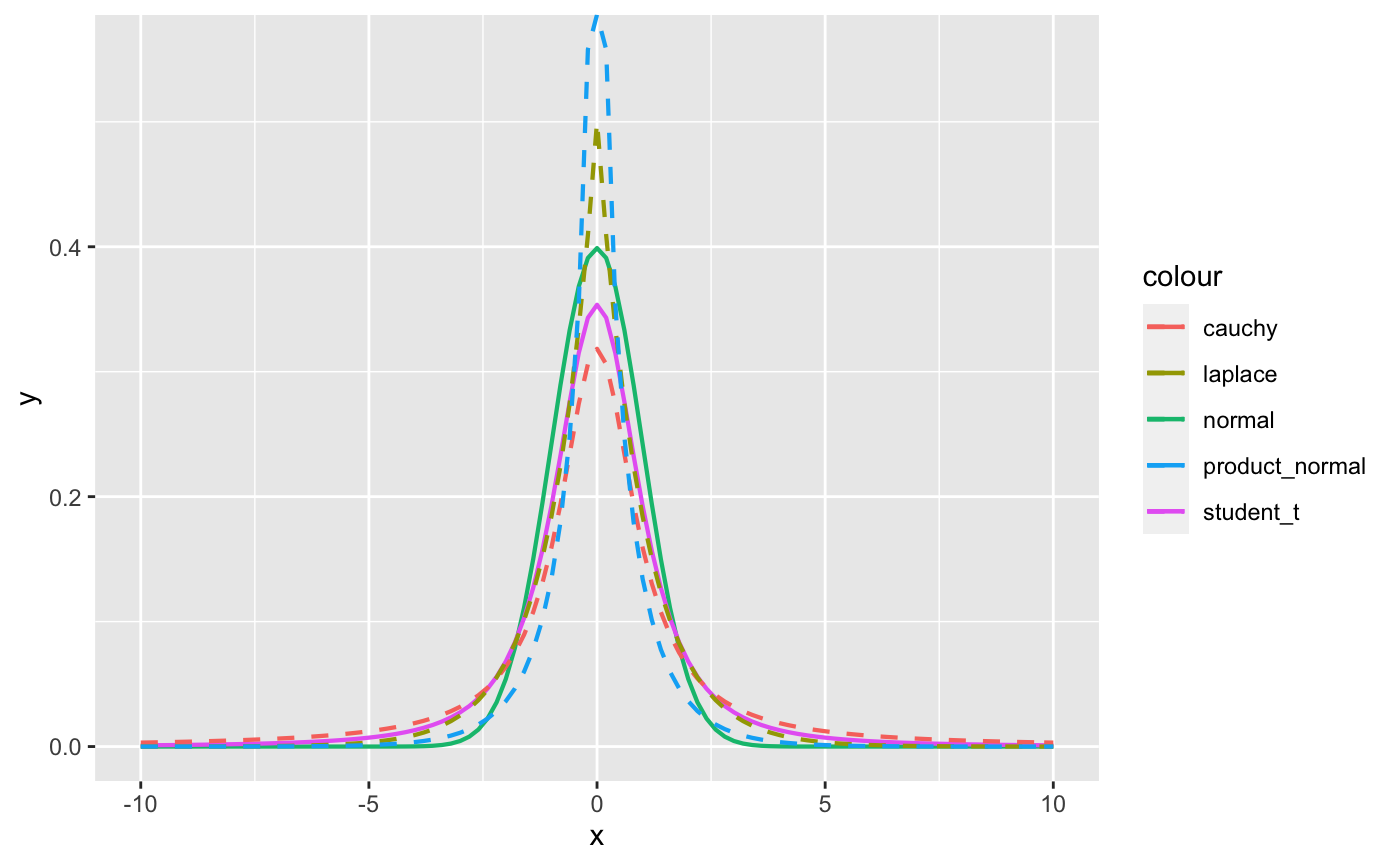# The student_t with df = 1 is the same as the cauchy
compare_priors(df_t = 1)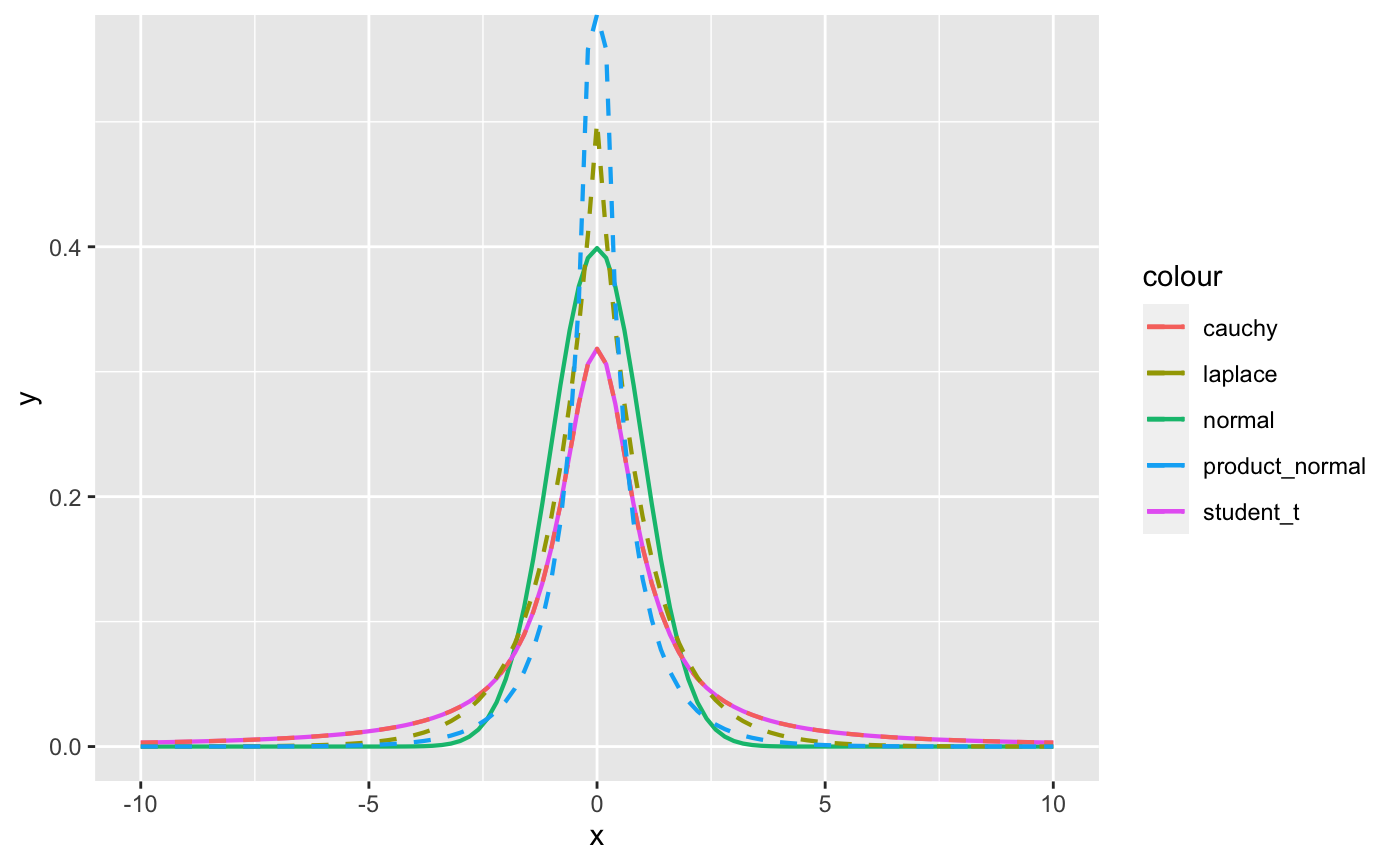# Even a scale of 5 is somewhat large. It gives plausibility to rather
# extreme values
compare_priors(scale = 5, xlim = c(-20,20))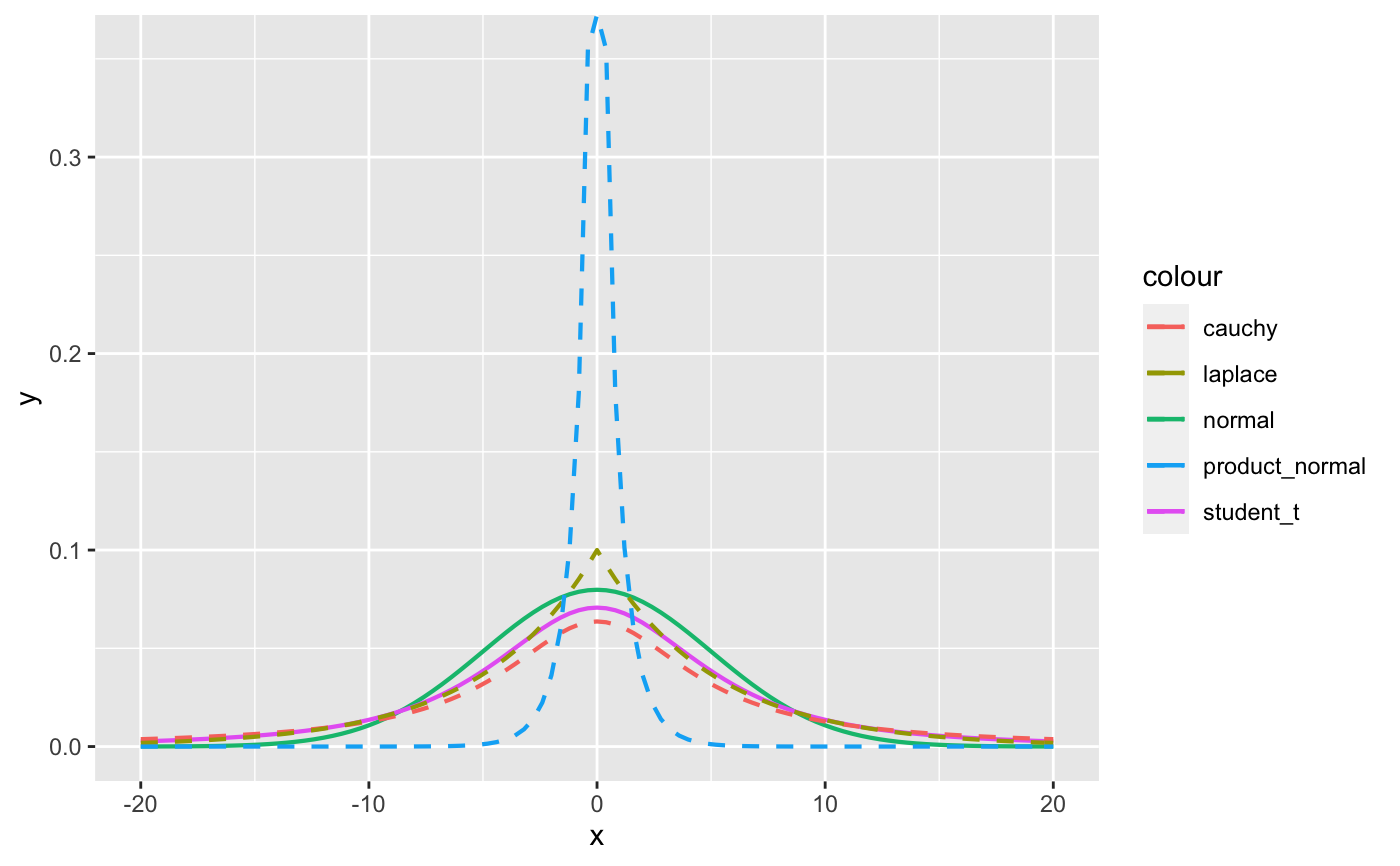# If you use a prior like normal(0, 1000) to be "non-informative" you are
# actually saying that a coefficient value of e.g. -500 is quite plausible
compare_priors(scale = 1000, xlim = c(-1000,1000))